# Logarithms – divide large numbers traditionally

Before the early 1970’s; before students had calculators:

It was incredibly difficult when dividing large numbers not to make a mistake.

For example 82,310 ÷ 162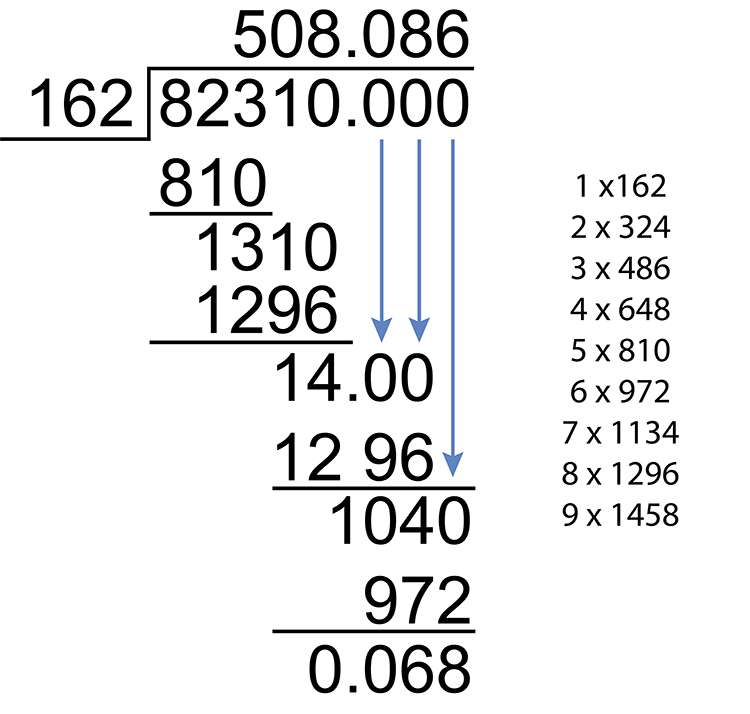508.086 remainder 0.068

Seriously try this divide and see if you make a mistake (for clarity we left out the subtraction workings out).

## Logarithms Divide

Mathematicians found an easier way.

They subtracted powers (indices/exponents), and subtracting is a lot easier.

From our knowledge of indices:

a^mdiva^n=a^m/a^n=a^(m-n)

Or 10^3 ÷ 10^2 = 10^1

((10times 10 \times 10))/(10\ times 10) = (10)

Here is the example again:

82310 ÷ 162

Is the same as 10^4.9155 ÷ 10^2.2095

Subtract the indices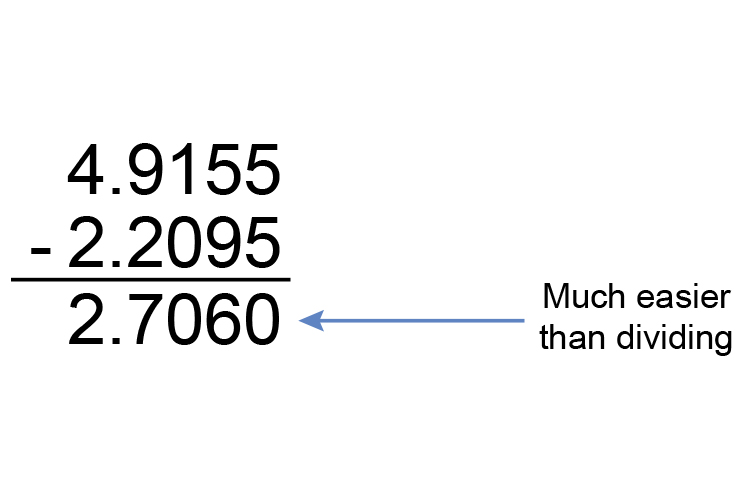This equals 10^2.7060

And 10^2.7060 = 10^2\times 10^0.7060

= 100\times 5.082

= 508.2

(see below for explanation)

Explanation

So how did we convert 82310 ÷ 162 into 10^4.9155 ÷ 10^2.2095

We use log tables            (see reference log tables above)

First look up 82310

Logs only work between 1 and 10

And so 82310 = 10^4\times 8.231

So we look up 8.231

Here is part of the log table: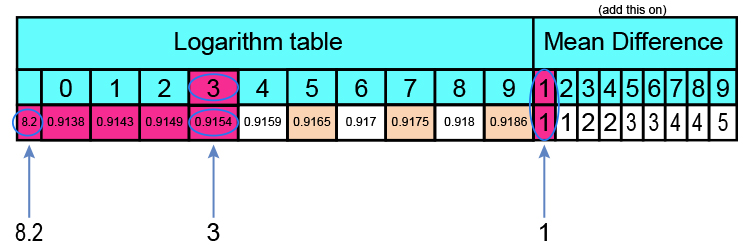0.9154 + 1 (to the last digit) = 0.9155

Log table of 8.231 = 0.9155

So 8.231 = 10^0.9155

Therefore:  82310 = 10^4\times 8.231

= 10^4\times 10^0.9155

= 10^4.9155 (adding indices)

Now look up 162

Logs only work between 1 and 10

And so 162 = 10^2times1.62

So we look up 1.62

Here is part of the log table:

(Reference log tables/reference anti-log tables above)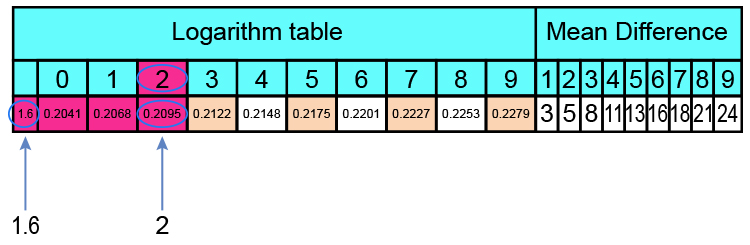Log table of 1.62 = 0.2095 which is spot on

So 1.62 = 10^0.2095

Therefore: 162 = 10^2times1.62

= 10^2\times 10^0.2095

= 10^2.2095 (adding indices)

So 82310 ÷ 162 is the same as 10^4.9155 ÷ 10^2.2095

Subtract the indicesThis equals 10^2.7060

## The antilog tables

So how do we convert 10^2.7060 back to a normal number?

We use antilog tables                                    (see reference antilog tables above)

Antilog 10^2.7060

Antilogs only work between 0 and 1

So 10^2.7060 = 10^2\times 10^0.7060

10^2 = 10\times 10 = 100

And we must look up 0.7060

Looking up 10^0.7060

Here is part of the antilog table: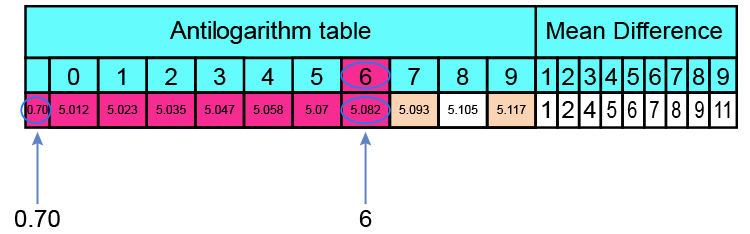Antilog table of 0.7060 = 5.082

So 10^0.7060 = 5.082

Therefore 10^2.7060 = 10^2\times 10^0.7060

= 100times5.082

= 508.2